﻿ 20-sim webhelp > Library > Iconic Diagrams > Mechanical > Rotation > Components > brake

# brake

## Library

Iconic Diagrams\Mechanical\Rotation\Components

C

V

CV

SCVS

LuGre

## Use

Domains: Continuous. Size: 1-D. Kind: Iconic Diagrams (Rotation).

## Introduction

The brake models represent a disk brake or other type of brake where a rotation is stopped by applying a friction force. The amount of friction depends on the normal force that is applied and the friction function that is used. The normal force is given by the input signal Fn. The brake is mounted to the fixed world.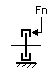The model has only one initial port p defined. Because any number of connections can be made, successive ports are named p1, p2, p3 etc. 20-sim will automatically create equations such that the resulting torque p.T is equal to the sum of the torques of all connected ports p1 .. pn. The angular velocities of all connected ports are equal to p.omega.

p.T = sum(p1.T, p2.T, ....)

p.omega = p1.omega = p2.omega = ....

Due to the use of normal force, the brake models all have a fixed torque out causality. The constitutive equations are therefore described as:

p.T = Fn * f(p.omega);

with f the friction function.

## Description - C

This model represents a brake with braking force described as coulomb friction. The brake is mounted to the fixed world. The amount of friction depends on the normal force that is applied:

p.T = Fn*mu_c*tanh(slope*p.omega);

Fn: normal force (given by the input signal Fn)

mu_c: the coulomb friction coefficient

slope: the steepness of the coulomb friction curve.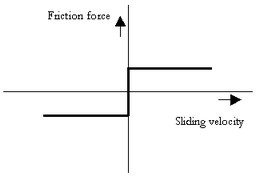## Interface - C

 Ports Description p[any] Any number of connections can be made (Rotation). Causality Fixed torque out Input Fn Normal force [N] Parameters mu_c slope Coulomb friction coefficient [m] Steepness of Coulomb friction curve [s/rad]

## Description - V

This model represents a brake with braking force described as viscous friction. The brake is mounted to the fixed world. The amount of friction depends on the normal force that is applied:

p.T = Fn*mu_v*p.omega;

Fn: normal force (given by the input signal Fn)

mu_v: the viscous friction coefficient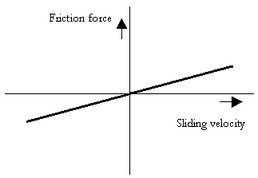## Interface - V

 Ports Description p[any] Any number of connections can be made (Rotation). Causality Fixed torque out Input Fn Normal force [N] Parameters mu_v Viscous friction coefficient [m.s/rad]

## Description - CV

This model represents a brake with braking force described as coulomb plus viscous friction. The brake is mounted to the fixed world. The amount of friction depends on the normal force that is applied:

p.T = Fn*(mu_c*tanh(slope*p.omega) + mu_v*p.omega);

Fn: normal force (given by the input signal Fn)

mu_v: the viscous friction coefficient

mu_c: the coulomb friction coefficient

slope: the steepness of the coulomb friction curve.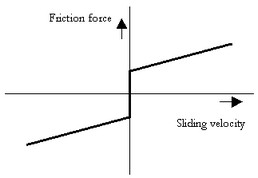## Interface - CV

 Ports Description p[any] Any number of connections can be made (Rotation). Causality Fixed torque out Input Fn Normal force [N] Parameters mu_v mu_v slope Viscous friction coefficient [ms/rad] Coulomb friction coefficient [m] Steepness of Coulomb friction curve [s/rad]

## Description - SCVS

This model represents a brake with braking force described as static plus coulomb plus viscous plus Stribeck friction. The brake is mounted to the fixed world. The amount of friction depends on the normal force that is applied:

p.T = Fn *

(( mu_c + (mu_st*abs(tanh( slope*p.omega )) - mu_c)

* exp( -((p.omega / v_st)^2 )) ) * sign(p.omega)

+ mu_v * p.omega);

Fn: normal force (given by the input signal Fn)

mu_s: the static friction coefficient

mu_v: the viscous friction coefficient

mu_c: the coulomb friction coefficient

slope: the steepness of the coulomb and static friction curve.

v_st: the characteristic Stribeck velocity.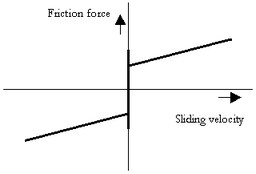## Interface - SCVS

 Ports Description p[any] Any number of connections can be made (Rotation). Causality Fixed torque out Input Fn Normal force [N] Parameters mu_s mu_v mu_c slope v_st Static friction coefficient [m] Viscous friction coefficient [m.s/rad] Coulomb friction coefficient [m] Steepness of Coulomb friction curve [s/rad] Characteristic Stribeck velocity [rad/s]

## Description - LuGre

This model represents a brake with braking force described by the LuGre friction model. The brake is mounted to the fixed world. The amount of friction depends on the normal force that is applied:

p.T = FN*f_lg(p.omega);

Fn: normal force (given by the input signal Fn)

f_lg: the LuGre friction model

## Interface - LuGre

Ports

Description

p[any]

Any number of connections can be made (Rotation).

Causality

Fixed Torque out

Input

Fn

Normal force [N]

## Parameters

mu_c

mu_s

mu_v

v_st

mu_k

Coulomb friction coefficient

Static friction coefficient [m]